##### Scripta cookbook

Please go to the introduction

 Note Be sure to select S in the toolbar, upper left to view source.

## About the cookbook

This cookbook contains many short recipes which illustrate how to accomplish various things in Scripta.

The recipes should be viewed using the X option in the control bar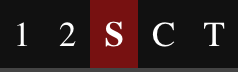which you will find on the upper left. By selecting S, you can view the source and rendered text simultaneously. Source on the left, rendered text on the right:

Source text ⇒ Rendered text

By comparing the two, you will quickly learn what you need to know. Begin with basic recipes, then select the recipes that are relevant to what you are writing.

To create a new section, you need to be in the Editor mode. Then click on the "+" button, above left in the toolbar.

 Note Be sure to select S in the toolbar, upper left, to view source.

## Basic recipesNote: This is only a test!

$a^2 + b^2 = c^2$

1. Orange Juice

2. Milk

3. Cereal

I read the New York Times every day.

To learn how to upload and use images, see the media section of the User Guide

## Lists

• Oranges

• Apples

• Pears

Fruit
• Oranges

• Apples

• Pears

1. Oranges

2. Apples

3. Pears

Fruit
1. Oranges

2. Apples

3. Pears

### More levels

Nature
1. Plants

1. Sunflowers

2. Sequoias

3. Dandelions

2. Animals

1. Carnivores

1. Lions

2. Tigers

2. Herbivores

1. Elephants

2. Rabbits

See the Asciidoctor Documentation for more information.

## Tables

### Simple table

 Eggs one dozen Potatoes twelve pounds Milk three quarts

 Eggs 12 0.56 $6.72 Potatoes 9 lb 0.45$4.05 Milks 3 quarts 1.89 $5.67 . ### Table with a header Description Quantity Unit Price Price Eggs 12 0.56$6.72

Potatoes

9 lb

0.45

$4.05 Milk 3 quarts 1.89$5.67

-

Description Quantity Unit Price Price

$16.44 Eggs 12 0.56$6.72

Potatoes

9 lb

0.45

$4.05 Milk 3 quarts 1.89$5.67

The source code
```[cols="<,^,>,>", options="header,footer"]
|===
| Description | Quantity | Unit Price | Price
| Eggs | 12 | 0.56 | $6.72 | Potatoes | 9 lb | 0.45 |$4.05
| Milk | 3 quarts | 1.89 | $5.67 | | | |$16.44
|===```

## Code

Use syntax coloring to better communicate code.

Sieve of Eratosthenes 
``````def eratosthenes(end, start=2, return_boolean=False):

# Finds all primes < `end`.

primes = []
if end < start or end < 2:
return []
is_prime = [True for i in range(end + 1)]
is_prime = is_prime = False
for i in range(2, end + 1):
if not is_prime[i]:
continue
if start <= i <= end:
primes.append(i)
j = i * i
while j <= end:
is_prime[j] = False
j += i
if return_boolean:
return primes, is_prime
return primes``````

## PDF files

Don’t create the text below by hand — follow the directions in the aside.

## Placing images

Please view this also using the S button: view the "aside".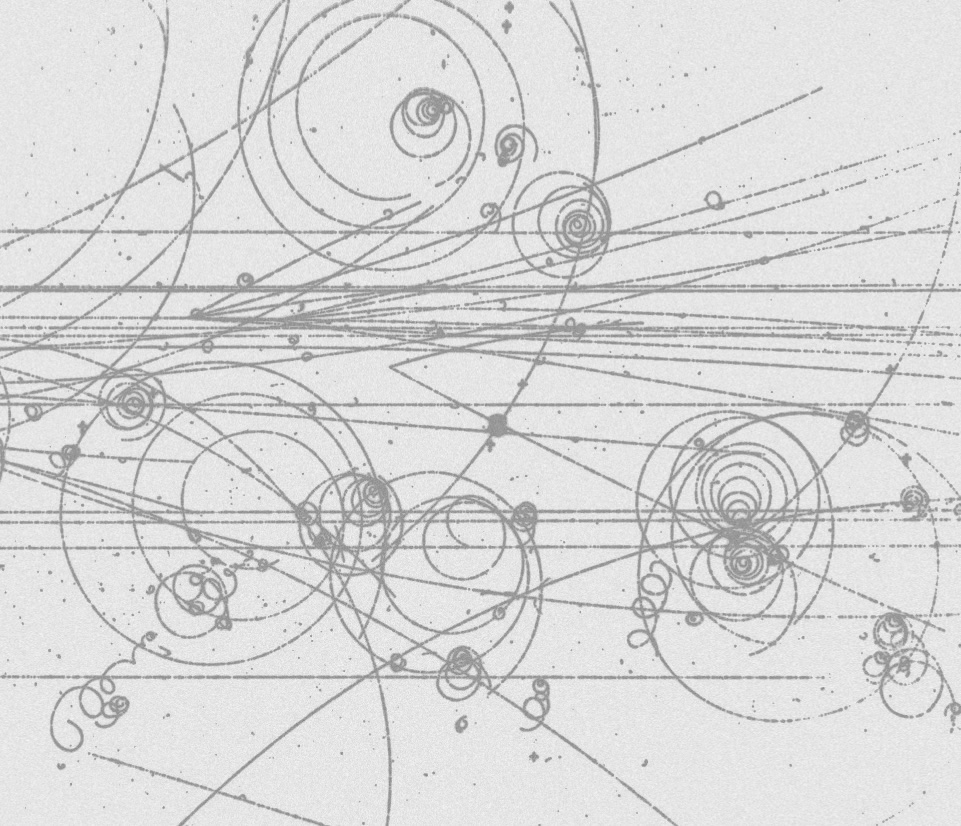## Linking to images and video

Images

If an image exists somewhere on the internet, you can link to it rather than uploading it to Scripta. Here is the model:

image ::URL[]

where URL is something like `http://whatever`. Thre is no space between 'image' and '::'Figure 1. is this a kind of robin?

You can also format the images in various ways, as in the image on the right. Here we used `float`. Another useful word is `align`. Notice also that we put a caption on this image. i you are using the X option (see upper left), you see how this is done.

The same method works for YouTube video;

You can also set the width and height;

video ::URL[youtube, width=500px, height=279px]

You will want to preserve the width-to-height ratio — the so-called aspect ratios. For the bird video, I did this as follows. First, I measured the YouTube video on my screen: 30.5 cm wide and 17 cm high. The aspect ratio is $30.5/17 = 1.794$. If I want to make the vide 500 pixels wide, I should make it $500/1.794 = 279$ pixels tall. As a check, we compute the ratio $500/279 = 1.792$ — close enough!

This reference has some good historical information.

Vimeo

The same principles apply:

Source; vimeo

*So the aspect ratio is $26/11 = 2.363$. For a 500 pixel wide video the height is $500/2.363 = 212$.

Geeky stuff about aspect ratios

The aspect ratio for old-style television is 4:3, and for HD it is 16:9. The "standard" ratio closest to the bird video above is 5:3. This fact can be deduced from the method of continued fractions or from the following simpler methid. Multiply the ratio 1.794 by the integers 2, 3, etc until you get a number close to an integer, as in the table below.

Multiplier Product Value Rounded Error

5

$5\times1.749$

8.97

9

$-0.03$

2

$2\times1.749$

3.588

4

0.588

3

$3\times1.749$

5.382

5

0.382

4

$4\times1.749$

7.176

7

0.176

Finding the aspect ratio

We conclude that aspect ratio is 9:5

## Mathematics

Use standard LaTeX syntax for inline and displayed mathematical text.

Inline formulas: $a^2 + b^2 = c^2$

Displayed formulas $\int_{-\infty}^\infty e^{-x^2/2} dx = \sqrt{2\pi}$

Theorems

Use Asciidoc-LaTeX environments for theorems.

Theorem 1.
There are infinitely primes
Theorem 2.
The series $\sum_{n=1}^\infty \frac{1}{n}$ is divergent

The text `oresme` after the hash mark is a label for cross-referencing, as in the example

I refer you to Theorem 2.

Environments in general. Instead of saying `[env.theorem]`, you can say `[env.lemma]`, `[env.definition]`, etc. These environments are numbered separately.

Equation environment. This is one of the special environments, treated differently from the others:

 $\sum_{n=1}^\infty \frac{1}{n} = \infty$

If the environment has a label, it is numbered:

 $\sum_{n=1}^\infty \frac{1}{n^2} = \frac{\pi^2}{6}$ (1)

We can then refer to it like this:

The series 1 is extremely cool!

Equationalign environment

 $\begin{split} a &= bc \\ b &= x + y \\ c &= x - y \\ a &= x^2 - y^2 \end{split}$

## Teaching

Click on the headings Hint and Answer below to reveal the hidden text. Click again to conceal it.

Question 1.
Who is buried in Grant’s tomb/
Hint
General Ulysses S. Grant
Question 2.
What is the length of the hypotenuse of a right triangle whose base is 3 meters and whose altitude is 1 meter?
Hint
Use the Pythagorean theorem, $a^2 + b^2 = c^2$
Solution
Since $3^2 + 1^2 = c^2$, $c = \sqrt{10}$.

## Bibliography

The Cyberiad [cyb], reviewed in [cyb2], recounts the adventures of the robots Trurl and Klaupicius as they explore the universe, encountering all manner of life-forms.

Bibliography

## Index

Suppose you are writing a text about animals:

```The Aardvark is a strange animal with an
equally strange name ...```

To add "Aardvark" to the index, change your text to this:

( ( (Aardvark) ) ) The Aardvark is a strange animal with an equally strange name …​

Here is the long form of an index mark:

( ( (Brontosaurus, diet) ) ) The diet of the brontosaurus consisted of …​

The index works only in the compiled view. Also, in order to instruct Scripta to make the index, you must add the code

`make_index: yes`

to your document preferences. To edit your document preferences, click on the triple-gear icon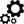.

## Glossary

You can mark terms for a per-section glossary like this: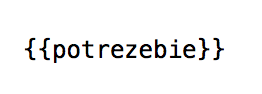In this case, the word potrezebie is inserted in the glossary that you will find at the end of this section. In the main text, you would find potrezebie set in a dark red color. Glossary terms link to their entry in the glossary list and vice versa.

You may also mark a glossary term like this: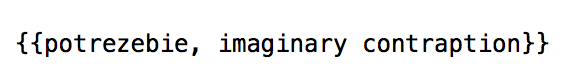In this case, the phrase "imaginary contraption" appears in the glossary as explanatory text for the term "potrezebie." (We do note, by the way, that there was a time in the previous millennium in which the term potrezebie appeared in certain forms of humorous litereature).

To instruct Noteshare to display the glossary, put the code `:glosssary:` somewhere in the section. The glossary will appear at the end of the section.

Glossary

 glossary an alphabetical list of significant words potrezebie imaginary contraption

1. Source: Github